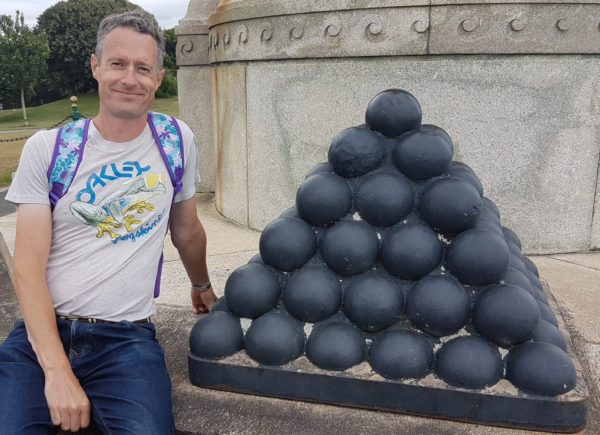07899 756585

# House of Maths School Workshops Primary & Secondary in Dorset & South - SQUARE PYRAMIDAL NUMBERS

### COUNTING CANNONBALLS

This pyramid of cannonballs appears at the foot of the Armada Monument on Plymouth Hoe. It consists of six layers: a single cannonball at the apex, resting on a square of 4 cannonballs, resting on a square of 9 cannonballs, and so on for a total of 6 layers.A reasonably curious mathematician might wonder how many cannonballs there are in the entire pyramid (including the “hidden” ones you can’t see on the inside of the pyramid).

The answer is the sixth Square Pyramidal number – which I’ll call $P_6$

### THE-NOT-VERY-CLEVER-WAY:

To find the total we could just go from layer to layer, adding as we go:

$P_6 = 1^2 + 2^2 + 3^2 + 4^2 + 5^2 + 6^2$

$= 1 + 4 + 9 + 16 + 25 + 36$

$= 91$.

To put this another way:
The first Square Pyramidal Number consists of only the first layer, so 1 object.
Add the next layer and we have 5 objects in total.
The third layer adds another $3^2 = 9$ objects, making 14 in total.
The sequence continues: 1, 5, 14, 30, 55, 91, …

Counting layer by layer works ok for small pyramids like our n=6, but suppose you had a 45 layer pyramid, starting with one at the apex and continuing for 44 more layers (with $45^2 = 2025$ cannonballs on just the base layer). That’s a lot of balls! – too many to count layer by layer. So now what?

### TWO CLEVER WAYS TO FIND THE SEQUENCE:

1. Visit the Online Encyclopaedia of Integer Sequences (OEIS); type the first few terms in and the OEIS will not only recognise the sequence but will give you the first 45 terms. Magic! Why not go ahead and try it now?
2. As the nth Square Pyrmaidal Number is the sum of the first n square numbers, you can use this formula for the sum of the first n square numbers:

### $P_n = \sum_{i=1}^{n} i^2 = \frac{1}{6}n(n+1)(2n+1)$

The $\sum$ is apronounced sigma and means “add all the terms together”. The proof (by induction) of this formula is well-known to any student of A-level Further Maths.

When n=6 our formula gives $P_6 = \frac{1}{6} \times 6 \times 7 \times 13 = 91$ – the same answer we found earlier. Try setting n=45 and comparing the answer with the 45th term from the OEIS database.

### ARE THERE OTHER TYPES OF PYRAMIDAL NUMBERS?

Yes! Other related sequences include the Tetrahedral numbers (given by the sum of the first n triangular numbers), and also the pentagonal, hexagonal or even higher order pyramidal numbers. Head over to the OEIS and if you can figure out the first few terms, the database will give you the next few, plus some interesting facts.

NOTIFY ME OF NEW POSTS BY EMAIL (approx one a month):

[mc4wp_form id=”399″]